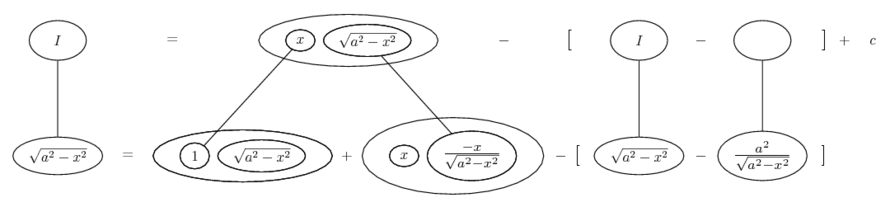# Thread: help with integration

1. ## help with integration

hello,

i've been in this question for ages and can't seem to crack it.

I need to substitute a suitable circular of hyperbolic fn so that I may find

a) integr[(a^2-x^2)^1/2]
b) integr[(a^2+x^2)^1/2]
c) ntegr[(x^2-a^2)^1/2]

I am looking at the first, and I think x=asinu => dx = acosu du

so (a^2-x^2)^1/2 = (acosu)^2 = [(a^2)/2] [cos2u+1]

and integr[cos2u+1] = [(sin2u)/2] + u + c_1

and subbing for u (this is one of my problems) I get my solution

= [(a^2)/2] {[(sin2arcsin(x/a))/2] + arcsin(x/a)} + c

I can also get it to

= (ax/2)[(pi/2) -a ) + (a^2/2)arcsin(x/a) +c

but i really want to get my book's answer which is

(1/2) { [ x(a^2 - x^2)^(1/2) - (a^2) arcsin(x/a)]}

please help me, I feel so fed up with trying this one question i have honestly been at it for hours!

2. The first is by parts (integrating 1 and differentiating the integral), re-writing

$\displaystyle \frac{x^2}{\sqrt{a^2 - x^2}}$

as

$\displaystyle \frac{a^2 - (a^2 - x^2)}{\sqrt{a^2 - x^2}}$

Will post a pic. The others look similar.

Edit: Just in case a picture helps...... where...is the product rule for differentiation (top to bottom).

In balloon world, integration by parts is a matter of filling out the product rule shape usefully, but starting in one of the bottom corners rather than (as with differentiation) the top one... and then subtracting whatever it's necessary to subtract on the bottom row, in order to keep the lower equals sign valid.

One blank to fill in the top row, then solve the top row for I.

________________________________

Don't integrate - balloontegrate!

http://www.ballooncalculus.org/forum/top.php

3. what can I say!! i am really impressed (by how much help you have offered me). Thanks!#### Search Tags

integration